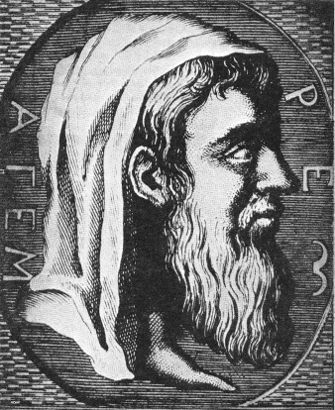#Euclid

Math Lair Home > Mathematicians > Euclid

"There is no royal road to geometry"
—EuclidEuclid (ca. 330–ca. 275 B.C.) was the first major scholar at the Library of Alexandria. His most important work was his Elements, a collection of the most important results of the previous three centuries of Greek mathematics. This book was divided into thirteen volumes, the first six of which dealt with plane geometry ("Euclidean geometry"), and the last seven dealing with solid (three-dimensional) geometry, number theory (including prime numbers, perfect numbers and more), and other topics.

More copies have been made of the Elements than of any other work, with the exception of the Bible. It was the world's primary geometry textbook for two millennia, until the 20th century. Clearly, it was a very important work. While Euclid worked out few of the theorems of Euclidean geometry himself, his Elements is very valuable in that it preserves the work of many Greek mathematicians that otherwise would have been lost.

For someone who had such an important influence on geometry, surprisingly little is known about Euclid. The best information we have is provided by Proclus, who wrote around 750 years after Euclid. Imagine that you were writing a biography about Fibonacci or some other mathematician who lived around 750 years ago; you would have no first-hand knowledge of that person's life. Similarly, Proclus would have had no direct knowledge of Euclid or his life. Proclus writes that Euclid worked in Alexandria during the reign of Ptolemy I; an anecdote exists involving him and a certain Ptolemy (which is the source of the quote at the top of the page), and he must have worked before the time of Archimedes (287–212 B.C.) because Archimedes cites Euclid, so the time frame that fits best for Euclid's work is during the reign of Ptolemy I (306–283 B.C.). The dates of Euclid's birth and death are entirely unknown.

Euclid began the Elements by defining various terms, and then stating five postulates. These form the basis of all his theorems. These well-known postulates are:

1. [It is possible] To draw a straight line from any point to any point.
2. [It is possible] To produce a finite straight line continuously in a straight line.
3. [It is possible] To describe a circle with any centre and distance.
4. That all right angles are equal to one another.
5. That, if a straight line falling on two straight lines makes the interior angles on the same side less than two right angles, the two straight lines, if produced indefinitely, meet on that side on which are the angles less than the two right angles.

Following the five postulates, Euclid also stated five "common notions", or axioms. The common notions are:

1. Things which are equal to the same thing are also equal to one another.
2. If equals be added to equals, the wholes are equal.
3. If equals be subtracted from equals, the remainders are equal.
4. Things that coincide with one another are equal to one another.
5. The whole is greater than the part.

From these postulates and common notions Euclid would derive a large number of theorems of plane geometry. This was a major accomplishment, and Euclid's work was the standard work on geometry for 2,200 years.

Elements was divided into 13 books. Books I to VI of Elements dealt with plane geometry, Books VII to IX dealt with number theory, Book X with irrational numbers, and Books XI through XIII with solid geometry. In all, there are around 465 propositions. While some of the propositions are Euclid's own, the majority had been previously proved by others. Euclid's main accomplishment was collecting these results so that they survived and organizing and presenting them systematically and formally.

In addition to the postulates and common notions stated by Euclid, there are several other assumptions in the Elements that modern mathematicians, devoted to precision and rigour, require be postulated instead of just assumed. For example, in the Elements, points are frequently determined by the intersection of a circle with a straight line or another circle, but there are no postulates defining under what conditions these will intersect. David Hilbert's Foundations of Geometry, first published in 1899, contains a set of 20 axioms or postulates for Euclidean geometry that eliminates such assumptions. Nonetheless, even though the Elements is incomplete by modern standards, it is still an amazing accomplishment considering that there is no other work in any other field of knowledge that stood the test of time for 2,200 years.

Euclid, like many other Greek mathematicians (Pythagoras being a particularly good example) would likely have held the idea of applying his mathematical theorems to practical use in contempt. Once, when asked how a certain theorem could be applied to practical use, he ordered his slave: "He must profit from learning; give him a penny". Interestingly, the word "geometry" is not to be found in the Elements. This might be because geometry meant "earth measurement" ("geo" = earth + "metria" = measurement) at the time. He likely didn't want people to think that his geometry was for practical use.

Sources used (see bibliography page for titles corresponding to numbers): 46.